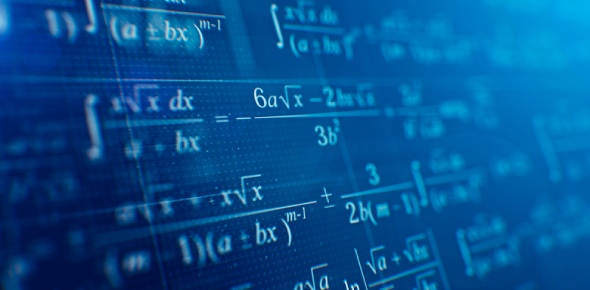# Mathematics Quiz On Basic Multiplication!

18 Questions | Total Attempts: 5912SettingsWhen it comes to multiplying figures be they large or small most people have a hard time doing the calculations without the use of calculators. Do you need some help solving some mathematical problems? Let us see if we can help you out by you trying out this test below and seeing if you can find the answer what happens when you multiply a number by six.

Related Topics
• 1.
6*8=
• A.

48

• B.

12

• C.

68

• 2.
6*2=
• A.

8

• B.

62

• C.

12

• 3.
6*6=
• A.

66

• B.

36

• C.

12

• 4.
6*9=
• A.

37

• B.

26

• C.

54

• 5.
6*7=
• A.

47

• B.

42

• C.

48

• 6.
6*5=
• A.

30

• B.

35

• C.

25

• 7.
6*3=
• A.

24

• B.

81

• C.

18

• 8.
6*1=
• A.

12

• B.

6

• C.

78

• 9.
6*4=
• A.

24

• B.

42

• C.

12

• 10.
6*11=
• A.

65

• B.

67

• C.

66

• 11.
6*10=
• A.

60

• B.

4

• C.

71

• 12.
6*12=
• A.

64

• B.

2

• C.

72

• 13.
6*8=
• A.

43

• B.

48

• C.

81

• 14.
6*2=
• A.

42

• B.

21

• C.

12

• 15.
6*9=
• A.

45

• B.

54

• C.

66

• 16.
6*7=
• A.

42

• B.

69

• C.

65

• 17.
6*11=
• A.

55

• B.

77

• C.

66

• 18.
6*12=
• A.

72

• B.

27

• C.

34